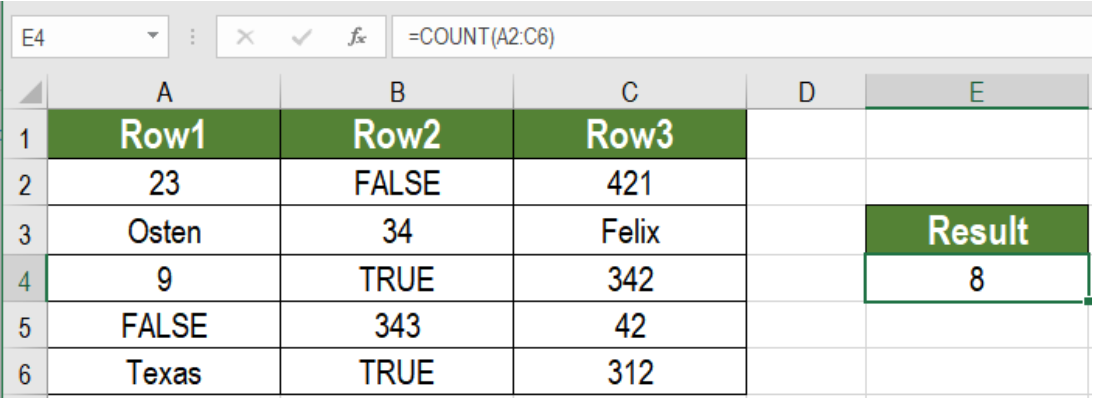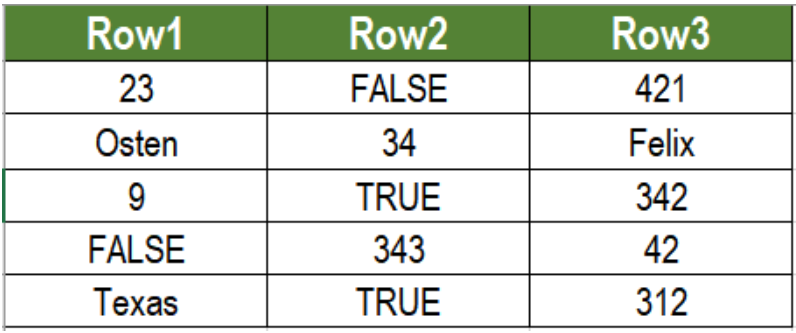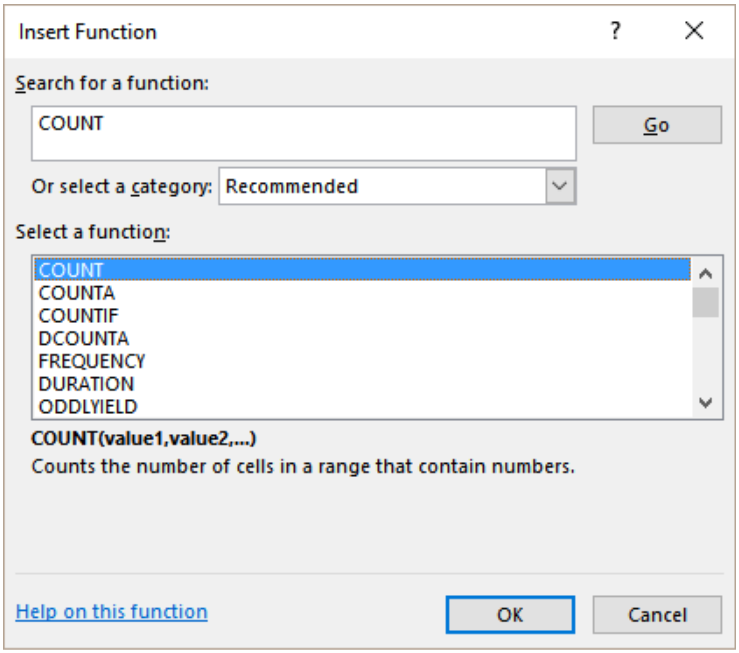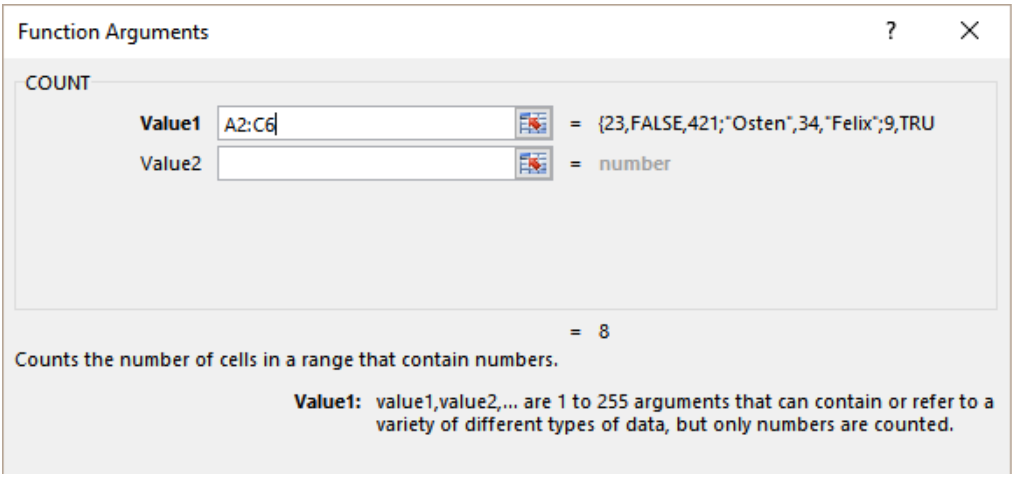Get instant live expert help with Excel or Google Sheets“My Excelchat expert helped me in less than 20 minutes, saving me what would have been 5 hours of work!”

#### Post your problem and you’ll get Expert help in seconds.

Your message must be at least 40 characters
Our professional Expert are available now. Your privacy is guaranteed.

# Count cells that contain numbers

There are simple ways to count cells containing numbers in Excel. We can do it using the COUNT function. It allows us to calculate numeric data regardless of the quantity of data on the spreadsheet. In this tutorial, we will learn how to count cells that contain numbers.Figure 1: Example of How to Count Cells that Contain Numbers

## Syntax

`=COUNT(range1, [range2..])`

• Range1
The range here is the range where we want to count the cells containing numbers. This value is required.
• [range2..]
More ranges to count numbers from. This value is optional. Excel can count up to 255 ranges.

COUNT is a fully automatic function. It calculates the result by counting cells in the range containing numbers.

## Setting up Data

In the following example, columns A, B and C contain some sample data. They contain text, numbers and logical values.Figure 2: The Sample Data

To count the cells that contain numbers:
Go to cell E4.

• Next, we need to click on Formulas>Insert Function. In the search box type COUNT and click Go.Figure 3: Searching the COUNT Function

• From the Select a Function box, we need to select COUNT and press Ok.
• Then, we need to input the range. In this case, it will be the range A2 to C6.Figure 4: Assigning the Range

• Press Ok.

Now we will have the formula `=COUNT(A2:C6)` in cell E4. This will show the result 8 in cell E4.

The COUNT function offers a fast and effective way to count the cells containing numbers in Excel.  This function is especially important when we need to count numbers fast and on time.

Most of the time, the problem you will need to solve will be more complex than a simple application of a formula or function. If you want to save hours of research and frustration, try our live Excelchat service! Our Excel Experts are available 24/7 to answer any Excel question you may have. We guarantee a connection within 30 seconds and a customized solution within 20 minutes.

### Did this post not answer your question? Get a solution from connecting with the expert.Another blog reader asked this question today on Excelchat:
Solution examplesI want to create a double bar graph from an existing pivot table, but excel won't let me simply add a new field for the column labels and manually put in numbers. What can I do?
Solved by C. F. in 18 minsI have many cells where value is seperated by commas e.g- 1,5,7,8,9 3,0,4,7,8,9,52, Just want to count total value, in the above case it should be 12
Solved by M. J. in 20 minsI have three spreadsheets with names of registered people for an event. I need to combine them and then filter so that I know if any of the people in sheet 1 are also listed on sheet 2 and sheet 3
Solved by C. A. in 40 minsI have a column of percentages (11 in a column). I want to have a percentage at the bottom of the column that tells me how many of the entries are = to 100%.
Solved by X. E. in 20 minsI have two lists of employers followed by 4 numbers. I then have another list with the same thing. The problem is that one has employers that the other doesn't so it doesn't line up to just sum each across. Is there a way I can find the sums without having to manually count?
Solved by A. A. in 20 mins## Subscribe to Excelchat.coAnother blog reader asked this question today on Excelchat: